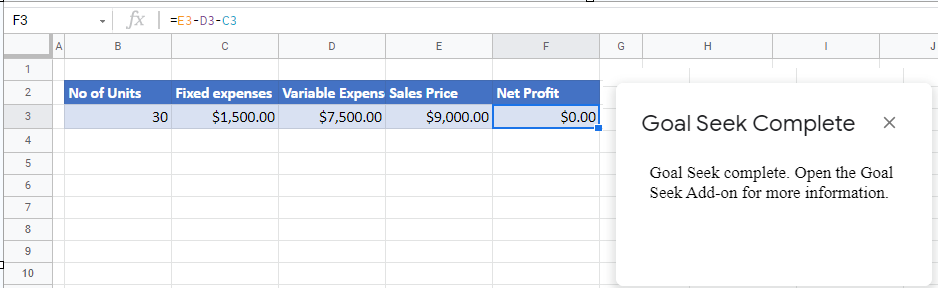# Calculate Break-Even Analysis Point in Excel & Google Sheets

This tutorial demonstrates how to calculate a break-even point in Excel and Google Sheets.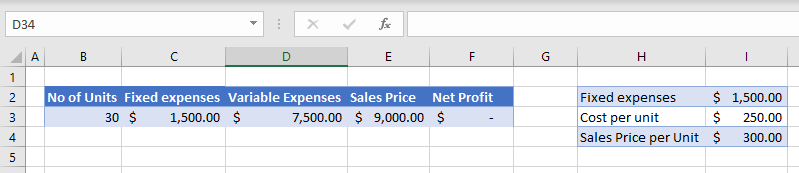A break-even analysis tells you how many units you need to sell at the sales price to get a profit of zero. This happens when a business’s total cost is equal to its total revenue – the “break-even” point.

## Calculate Break-Even Point

Say you’ve set up a worksheet with the number of units, the fixed expenses (costs that occur regardless of how many units you sell), the variable expenses (per unit to produce/supply one unit), and finally, the sale price.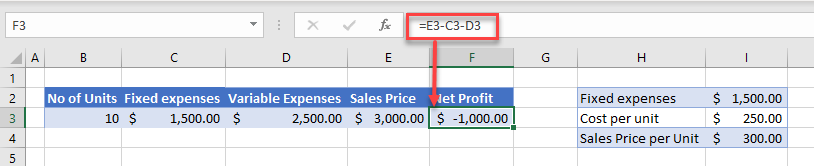Net Profit = (Unit Price × Number of Units) – Fixed Expenses – (Variable Expenses × Number of Units)

For this example, net profit for the sale of 10 units is \$300 per unit x 10 units minus \$1,500 minus \$250 per unit x 10 units. If you sell 10 units as shown above, you have a loss of \$1,000. To find the break-event point, find out how many units you need to sell for the net profit to be zero.

1. With cell F3 (Net Profit) selected, in the Ribbon, go to Data > What-If Analysis > Goal Seek.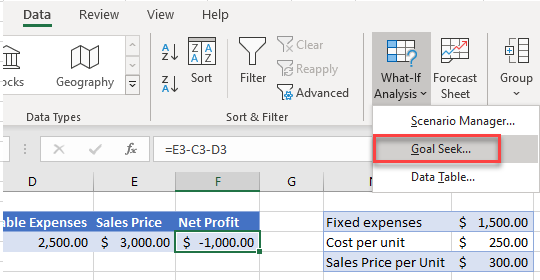1. Set three values:
• Set cell – This cell has to contain a formula and is the cell where the analysis sets to the target value. In this example, the formula in cell F3 calculates profit.
• To value – This is the target value for the Set cell. When finding the break-even point, always use a target value (i.e., Net Profit) of zero.
• By changing cell – This is the variable cell. Here, Goal Seek finds the value at which the variable (number of units) sets the formula cell to the target value (i.e., results in zero profit).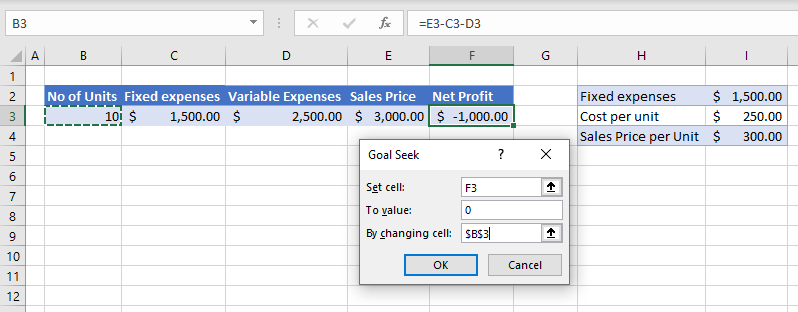1. Click OK to start the Goal Seek analysis. Since there are multiple variables, it may take a while!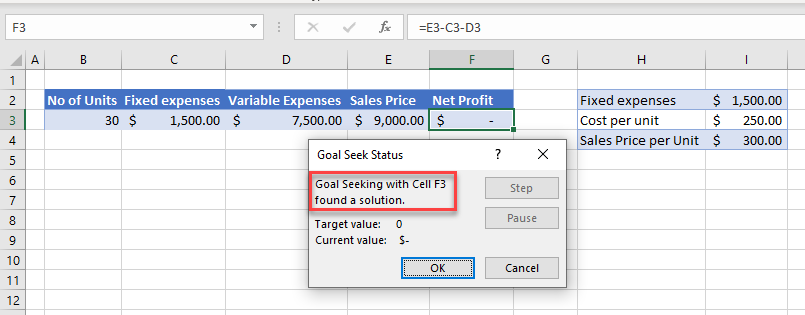1. Once a solution is found, click OK. In this example, the business breaks even when 30 units are sold.

## Calculate Break-Even Analysis Point in Google Sheets

1. In the Menu, go to Add-ons > Goal Seek > Open.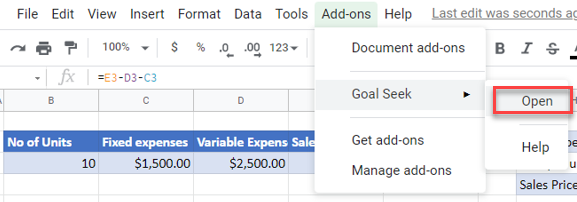1. Goal Seek opens on the right side of the screen. Set Cell refers to the calculated value (here, F3). In the To Value field, type in the target value (0). Finally, set the variable cell. This cell (B3, No of Units) is where Goal Seek’s result appears.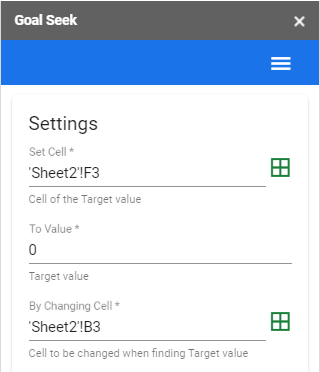1. You can adjust the Options to set the maximum iterations or the tolerance or time limit values.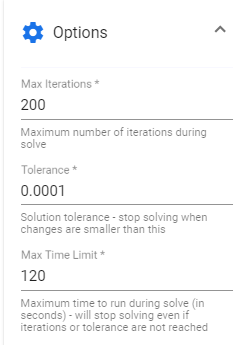1. Click Solve to run the analysis.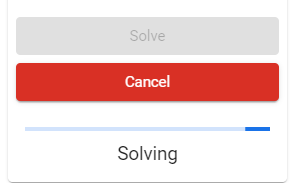1. Once a solution is found, a Goal Seek Complete message pops up, displaying the result onscreen.